Geometry

# Two Secants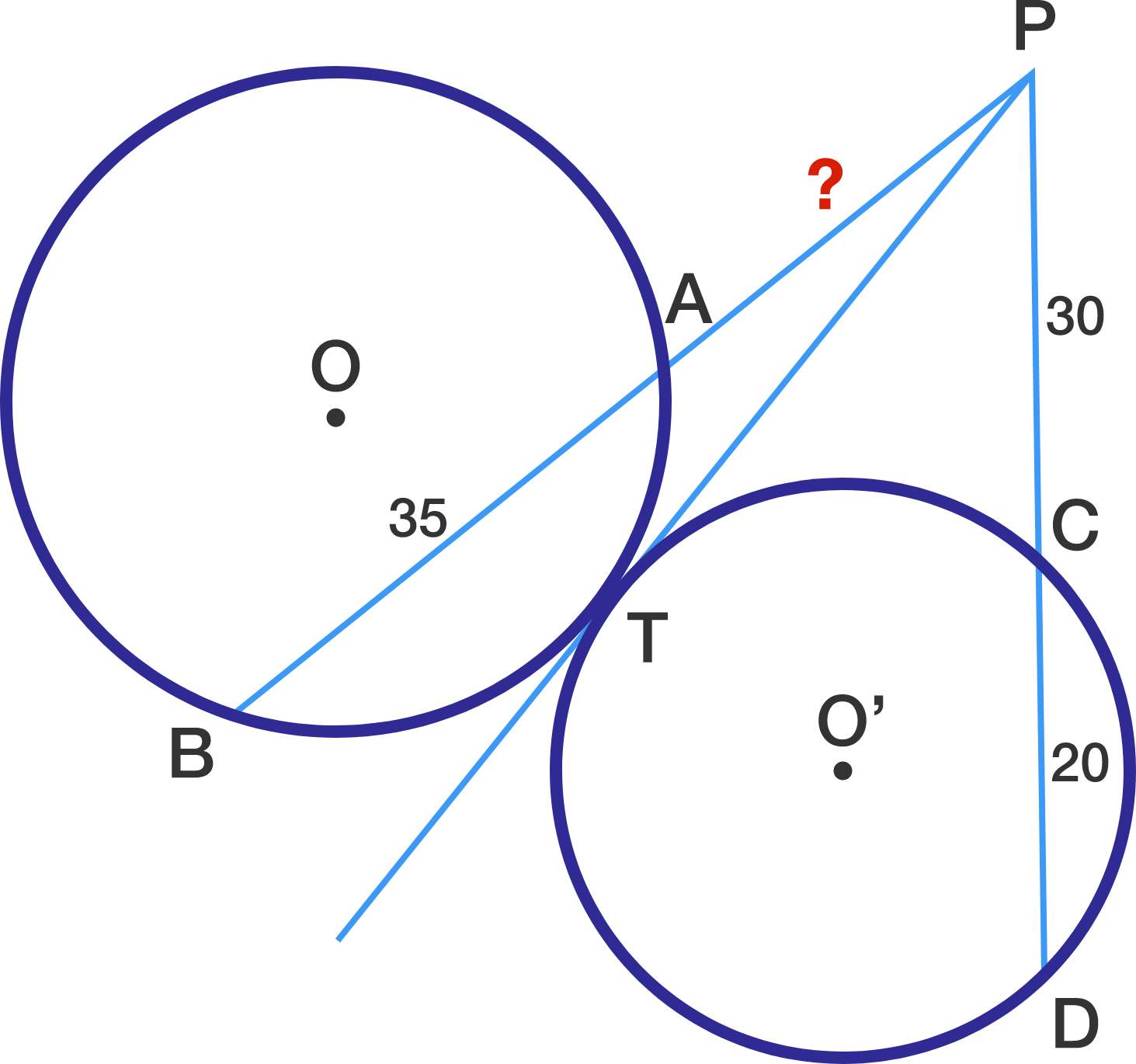In the above diagram, line segment $\overline{PT}$ is tangent to both circle $O$ and circle $O'.$ Given the following three lengths: $\lvert\overline{AB}\rvert = 35, \lvert\overline{PC}\rvert = 30, \lvert\overline{CD}\rvert = 20,$ what is $\lvert\overline{PA}\rvert?$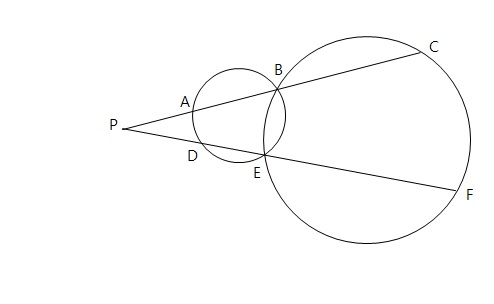In the above diagram, we are given the following four lengths: $\lvert \overline{PA} \rvert=25, \lvert \overline{AB} \rvert=35, \lvert \overline{PD} \rvert=30, \lvert \overline{EF} \rvert=70.$ Then what is the value of $\lvert \overline{BC} \rvert+\lvert \overline{DE} \rvert?$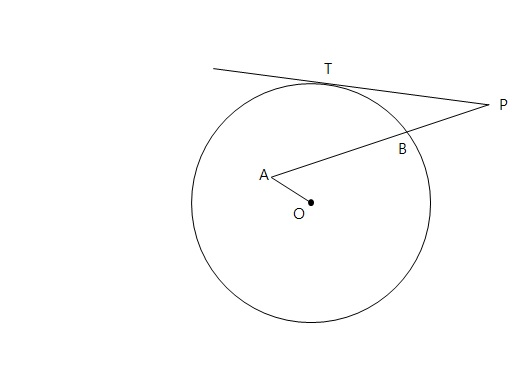In the above diagram, $\overline{PT}$ is a tangent line to circle $O$ which has radius $r.$ Given the following four lengths: $\lvert\overline{PT}\rvert = 48, \lvert\overline{PB}\rvert = 24, \lvert\overline{AB}\rvert = 40, \lvert\overline{AO}\rvert = 16,$ what is the value of $r^2?$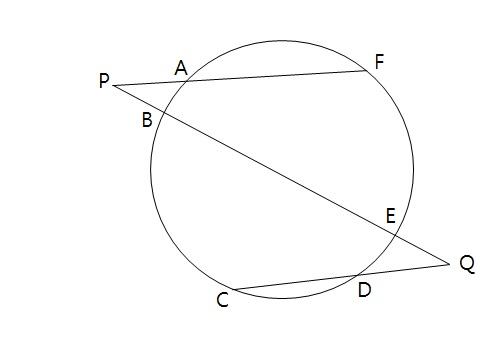In the above diagram, we are given the following three lengths: $\lvert \overline{AP} \rvert = 6, \lvert \overline{AF} \rvert = 13, \lvert \overline{DQ} \rvert = 5.$ If $\lvert\overline{PB}\rvert = \lvert\overline{QE}\rvert,$ what is $\lvert\overline{CD}\rvert?$

Note: The above diagram is not drawn to scale.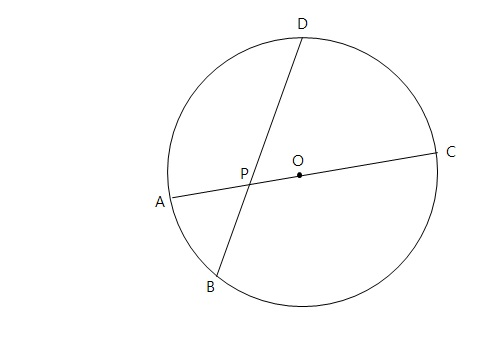In the above diagram, $\overline{AC}$ is a diameter of circle $O$ with radius $49.$ If $\overline{AC}$ intersects chord $\overline{BD}$ at $P$ and $\lvert\overline{AP}\rvert\ = 28, \lvert\overline{PD}\rvert\ = 56,$ what is $\lvert\overline{BP}\rvert?$

×